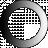# American Dictionary of the English Language

Dictionary Search

### Binary

BI'NARY, adjective [Latin binus, two and two.]

Binary arithmetic, the invention of Leibnitz, is that in which two figures only, 0 and 1, are used, in lieu of ten; the cypher multiplying every thing by two, as in common arithmetic by 10. Thus, 1 is one; 10 is two; 11 is three; 100 is four; 101 is five; 110 is six; 111, is seven; 1000 is eight; 1001 is nine; 1010 is ten. It is said this species of arithmetic has been used by the Chinese for 4000 years, being left in enigma by Fohi.

Binary measure, in music, is that used in common time, in which the time of rising in beating, is equal to the time of falling.

Binary number is that which is composed of two units.

BI'NARY, noun The constitution of two.